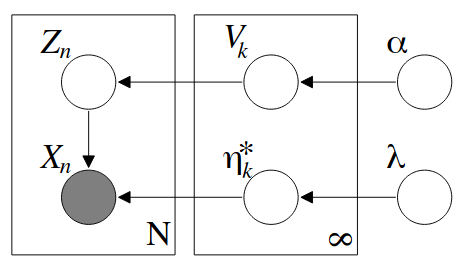# Rylan SchaefferAbout
Resume
Research
Learning
Blog
Talks
Teaching
Jokes
Kernel Papers

21 March 2021

# Variational Inference for Dirichlet Process Mixtures

by Blei, Jordan (Bayesian Analysis 2006)

## Research Questions

• How to perform variational inference in Dirichlet Process (DP) Mixture Model?

## Background

For a quick primer on Dirichlet processes and their use in mixture modeling, see my notes on DPs

## Approach

Assuming the observable data is drawn from an exponential family distribution and the base distribution is the conjugate prior, we have a nice probabilistic model:

1. Draw $$V_i \lvert \alpha \sim Beta(1, \alpha)$$. Let $$\underline{V} = \{V_1, V_2, ...\}$$
2. Draw parameters for the mixing distributions $$\eta_i^* \lvert G_0 \sim G_0$$, where $$G_0$$ is the base measure of the DP. Let $$\underline{\eta^*} = \{\eta_1^*, \eta_2^*, ...\}$$.
3. For the $$n= 1, ..., N$$ data point
• Draw $$Z_n \lvert \underline{V} \sim Multi(\pi(V))$$
• Draw $$X_n \lvert Z_n \sim p(x_n \lvert \eta_{z_n^*})$$In constructing the variational family, we take the usual approach of breaking dependencies between latent variables that make computing the posterior difficult. Our variational family is

$q(\underline{V}, \underline{\eta^*}, \underline{Z}) = \prod_{k=1}^{K-1} q_{\gamma_k}(V_k) \prod_{k=1}^K q_{\tau_k}(\eta_t^*)\prod_{n=1}^N q_{\phi_n}(z_n)$

where $$K$$ is the variational truncation of the number of mixing components and $$\{\gamma_k\} \cup \{\tau_k\} \cup \{\phi_n \}$$ are our variational parameters.

tags: dirichlet-process - variational-inference - mixture-models - bayesian-nonparametrics